### 1. Summary

The value effect is one of the most studied market anomalies [2-5]. The value effect or value premium refers to the tendency of stocks with lower valuation ratios to earn above average returns over the long run. For example, the cross-sectional variation of stock returns across countries can be partly explained by a global value factor . Such a value effect has been observed across many different markets, regions and sample periods .

In this paper, we use a combination of several common valuation measures to capture the value premium. We assess forecast measures of Earnings Yield, Book to Price, Cash Flow Yield, Sales to Price and Dividend Yield. All valuation measures are calculated on a 12-month forward basis using IBES estimates. We find a value premium that is economically significant using both absolute and relative measures of value. However, the ability to capture any value premium varies across valuation measures.

In terms of absolute measures of value, Earnings Yield is the most significant, followed by Cash Flow Yield, Sales to Price, Book to Price and finally Dividend Yield. Country relative measures of value are generally comparable to absolute measures of value in their ability to identify a value premium: the exceptions are Earnings Yield which is poorer and Sales to Price, which is superior to its absolute measure. Furthermore, when value is measured by Earnings Yield, Cash Flow Yield and Sales to Price, value effects are relatively persistent over time.

The strength and persistency of individual value effects and levels of correlation amongst individual valuation measures suggest that a combination of Earnings Yield, Cash Flow Yield and Sales to Price is appropriate. We illustrate the simulated historical performance characteristics of various composite value factors within the context of a set of illustrative indexes. Given the high correlation between Cash Flow Yield and Book to Price and the similarity of index level characteristics between indexes formed on two and three-factor composites, we prefer a three-factor composite value measure. Since our analysis is very much based “in sample”, this is a cautious choice which ensures greater factor diversity. This choice has the further advantage that our composite factor has a greater orientation towards factors that are less susceptible to manipulation (Cash Flow Yield and Sales to Price) than to those that are (Earnings Yield).

Index characteristics, including capacity, levels of diversification and the degree of exposure to the factor of interest are robust to the frequency and timing of the index rebalance. Rebalance frequency has a substantial impact on turnover. An annual rebalance minimises turnover; we make the pragmatic decision to rebalance annually in September order to synchronise index rebalances with reviews of the underlying index universe and other factor (Quality) indexes.

We construct a broad value index that includes all underlying index constituents. We subsequently form a narrow value index containing fewer stocks and displaying greater exposure to value and assess the robustness of performance outcomes. This increased exposure to value results in improvements in performance compared to a broad value index.

The paper is structured as follows: Section 2 assesses alternative measures of valuation from the perspective of signal strength, persistency and correlation.

Section 3 examines practical factor indexes based on individual valuation measures. A broad composite value index is examined in Section 4 in the context of an appropriate rebalance frequency. Section 5 examines the implications of forming a narrow composite value index. Section 6 concludes.

### 2. Regression analysis

In order to assess the pure factor performance characteristics of each value factor, we perform the regression analysis detailed in Appendix A. This analysis results in the return to each ideal factor mimicking portfolio and therefore avoids any dependence on the method of index construction chosen.

We examine constituents of the FTSE Developed universe, performing monthly cross-sectional regressions for the period March 2000 to December 2013. Table 1 shows the average ideal monthly factor returns and t-stats for each individual factor with and without the inclusion of industry and country membership as additional factors. An equally weighted regression scheme and monthly local price returns are used to form each cross-sectional regression.

Earnings Yield is the strongest and most significant factor in absolute terms, followed by Cash Flow Yield, Sales to Price, Book to Price and finally Dividend Yield. All industry relative measures result in lower absolute levels of significance and mean returns. All country relative measure results are comparable to the absolute factor outcomes with the exceptions of Earnings Yield which is poorer and Sales to Price which is superior to its absolute measure. This suggests that absolute value measures may be used for Earnings Yield, Book to Price, Cash Flow Yield and Dividend Yield, whilst a country relative measure is appropriate for Sales to Price. Throughout the rest of this document we adopt these measures.

Chart 1 displays the two year rolling average t-stats through time for each valuation factor. The graphic illustrates the significance of Earnings Yield, Cash Flow Yield and Sales to Price is relatively persistent through time. Although Book to Price is relatively stable, post early 2007 t-stats are mainly negative. Dividend yield is relatively unstable and insignificant throughout most of the period.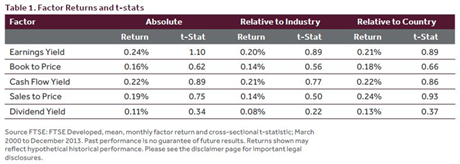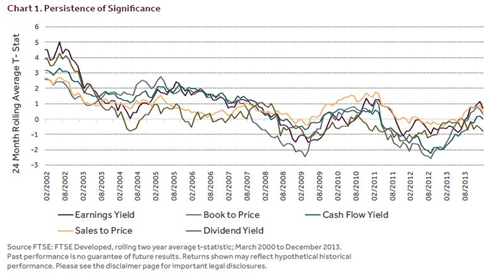Chart 2 shows the cumulative ideal factor return for each value factor. The performance of value in general has been flat post September 2007. However all factors apart from Dividend Yield capture the “value rally” post the September 2008 global financial crisis.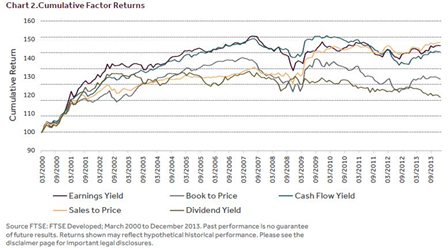Table 2 shows the correlation between monthly ideal factor returns. Note that Book to Price, Cash Flow Yield and Sales to Price are all strongly positively correlated to one another. Earnings Yield shows a weaker positive correlation with all factors, whilst Dividend Yield is only notably positively correlated with Earnings Yield.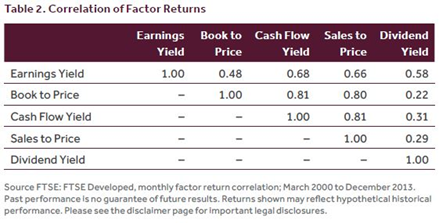Table 3 shows the cross-sectional correlation of raw value factor characteristics i.e. between the valuation measures themselves rather than between the factor returns of each valuation measure. This pattern is weaker, but similar to that observed for factor returns.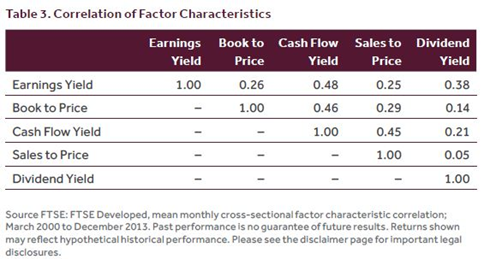### 3. Single factor indexes

In this section we create factor indexes premised on individual value factor characteristics. We employ the index construction techniques set out in ‘Factor Exposure Indexes – Index Construction Methodology’, FTSE (2014) . Briefly, we map a normalised valuation measure (Z-Score) to a score in the range of zero to one using a cumulative normal mapping. This score is combined with a stock’s weighting in an underlying index to determine individual stock weightings in the value factor index. The approach is tantamount to a factor tilt on an underlying index, where the strength (and number) of any tilts and the degree of diversification may be controlled.

Simulated performance figures in the remainder of this document are USD price returns for the period May 2000 to December 2013, where a monthly rebalance frequency is utilised for illustrative purposes only.

Table 4 shows the simulated historical performance figures of indexes that result from tilting away from an underlying market capitalisation weighted index towards each of the alternative value measures. We also show the resulting index level factor exposure (the weighted sum of individual stock value factor Z-Scores, see Appendix B) relative to the underlying universe and the degree to which each index loads on the ideal factor index (see appendix A for details of this calculation). Finally, we assess index capacity as measured by the weighted average capacity ratio (WCR) and consider the degree of diversification via the average Effective N through time (see Appendix B for both definitions).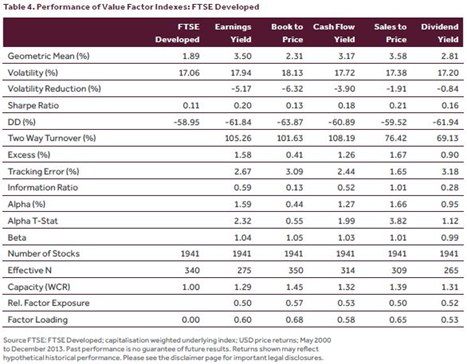Earnings Yield, Cash Flow Yield and Sales to Price have historically proven superior measures of value to Book to Price and Dividend Yield on most performance measures. However, a Book to Price value index is the most diversified (measured by Effective N) and the Dividend Yield value index exhibits substantially lower levels of two way turnover.

All tilted indexes achieve a similarly high loading on the relevant ideal factor index and exhibit substantial exposure to the factor of interest relative to the underlying index.

Chart 3 shows the simulated historical cumulative performance for each factor index relative to FTSE Developed through time.

Table 5 shows the simulated performance figures for a corresponding set of value indexes, where the factor tilt is applied to an equally weighted version of the FTSE Developed index.

As expected, the capacity of these indexes is lower than the market capitalisation tilted counterparts, whilst levels of diversification (Effective N) are superior. Each index continues to exhibit a high loading on the relevant ideal factor index and a superior factor exposure relative to the underlying index.

The relative performance of both sets of indexes is broadly similar. Relative performance is the result of the degree of factor exposure rather than underlying index weighting scheme.